Home > GHCN, trb > trb-0.02: Simple Latitudinal Zoning

## trb-0.02: Simple Latitudinal Zoning

2010 July 11

Introduction

Why use latitudinal zoning?

In version 0.01, I took a simple mean of the data set. But we know that the data set (weather stations) are not evenly distributed in space. There are many more stations in mid-latitudes than in high-latitudes. Many more in the northern hemisphere than in the southern hemisphere.

First lets look at an evenly distributed data set. In this case it doesn’t matter if we average all at once, over the rows first, or over the columns first. The complete data gives us the same mean value by all three methods.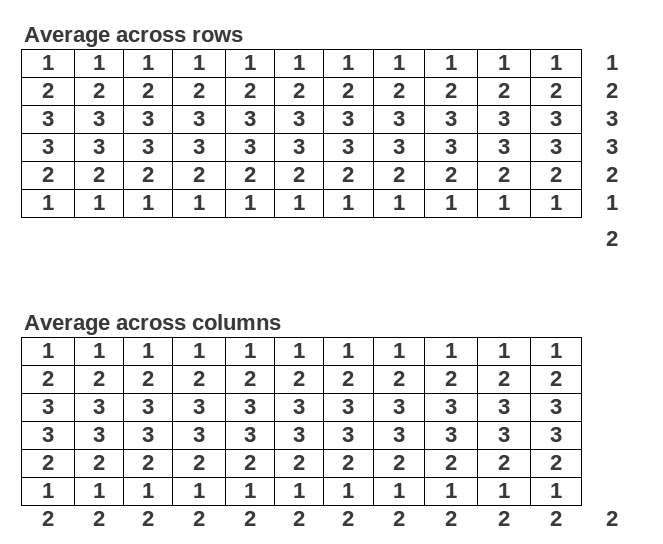But what if the data values and the missing data cells are not evenly distributed over the grid? Does the manner in which by which we average matter? Yup.The reason we have to average the each row first and then average the rows is because the data values are not uniform and the empty cells are not distributed randomly. The outer rows are more likely to have both lower values and empty cells. If we averaged by columns first, our averages would tend to be too high because of the biased distribution of empty cells in a grid in which the values depend on the row. By averaging over the row first, we can compensate for this non random distribution of empty cells.

Of course this works in this example because the data values show a strong dependence on the row. But we expect that in the real world too. Temperature is strongly dependent on the latitude, on the rows. So averaging by latitudinal zones makes some sense given the real world pattern of weather station distribution (sparse in the high latitudes, sparse in the southern hemisphere).

Code

I’ve hightlighted the new stuff.

```###############################################################################
# trb 0.02 : 2010 07 11 : Simple Latitudinal Bands
# trb 0.01 : 2010 07 10 : GHCN Simple Mean Average
# https://rhinohide.wordpress.com
###############################################################################

###############################################################################
###############################################################################
# ftp://ftp.ncdc.noaa.gov/pub/data/ghcn/v2/v2.mean
# ftp://ftp.ncdc.noaa.gov/pub/data/ghcn/v2/v2.temperature.inv
###############################################################################

#------------------------------------------------------------------------------
# Temperature File (GHCN formatted monthly means)
#------------------------------------------------------------------------------

# mm for 'monthly means'
mmwids=c(11,1,4,5,5,5,5,5,5,5,5,5,5,5,5)
mmcols=c("id","dupe","year","jan","feb","mar","apr","may","jun",
"jul","aug","sep","oct","nov","dec")

#------------------------------------------------------------------------------
# Inventory File
#------------------------------------------------------------------------------

# there are five occurrances of the pound hash in v2.temperature.inv
# R scripts chokes on reading those, remove them first

invcols <- c("id","name","lat","lon","alt","elev","ru","pop","terr","arid",
invwids <- c(11,32,6,8,5,5,1,5,2,2,2,2,1,2,16,1,5)

###############################################################################
# Set up arrays for latitudinal banding
###############################################################################

hindex=new.env(hash=TRUE)
hlat=new.env(hash=TRUE)
hlon=new.env(hash=TRUE)
for (i in 1:nrow(inv)) {
hindex[[as.character(inv[i,1])]] <- i
hlat[[as.character(inv[i,1])]] <- inv[i,3]
hlon[[as.character(inv[i,1])]] <- inv[i,4]
}

mmlat <- rep(NA,nrow(mm))
mmlon <- rep(NA,nrow(mm))

for (i in 1:nrow(mm)) {
mmlat[i] <- hlat[[as.character(mm[i,1])]]
mmlon[i] <- hlon[[as.character(mm[i,1])]]
}

bands <- c(90,60,30,0,-30,-60,-90)

###############################################################################
# Calculate Annual Mean Average
###############################################################################

#------------------------------------------------------------------------------
# set up the range of years to calculate
#------------------------------------------------------------------------------
#year0 <- min(mm[,3])
year0 <- 1880
year1 <- max(mm[,3])
range <- year1 - year0 + 1

#------------------------------------------------------------------------------
# initialize some vectors to hold annual results
#------------------------------------------------------------------------------
#lmean <- rep(NA, range -1)
#lcount <- rep(NA, range -1)
lyear <- rep(NA, range)
lmean <- matrix(NA,range,length(bands)-1)
lcount <- matrix(NA,range,length(bands)-1)

#------------------------------------------------------------------------------
# loop through the range of years
#------------------------------------------------------------------------------
for (i in 1:range) {

year <- i + year0 -1

#------------------------------------------------------------------------------
# loop through the latitude bands
#------------------------------------------------------------------------------

latlen = length(bands) -1
for (j in 1:latlen) {

# select the latitude out of the mm list first
# since lat data is not embedded in mm and we depend on symmetry with mmlt
lmax <- bands[j]
lmin <- bands[j+1]
idx=(mmlat<=lmax & mmlat>=lmin)
mmlt=mm[idx,]

# select the year out of the mmlt
idx=(mmlt[,3]==year)
mmyr=mmlt[idx,]

# calculate the annual means
# divide by 10 since means are stored as 10ths of T

# only use years with data for every month
lmean[i,j]=mean(rowMeans(mmyr[,4:15]),na.rm=T)/10

# use every year, even those with partial data
#lmean[i,j]=mean(rowMeans(mmyr[,4:15],na.rm=T),na.rm=T)/10

lcount[i,j]=nrow(mmyr)
}

lyear[i]=year
}

#------------------------------------------------------------------------------
# calculate the global land temperature
#------------------------------------------------------------------------------
ameans = rowMeans(lmean,na.rm=T)
mean(ameans, na.rm=T) # 10.48877

###############################################################################
# Display
###############################################################################

# plot some stuff
#png("trb-002-ghcn-lat-temp.png", width=600, height=400)
plot (ameans ~ lyear, type="l", xlab="Year", ylab="Temp (C)",
main="GHCN Simple Latitudinal Averaged Temps",
sub="https://rhinohide.wordpress.com (trb-0.02)")

png("trb-002-zone-1.png", width=600, height=150)
par(mar=c(2, 2, 2, 1))
plot(lmean[,1] ~ lyear, ylab="Temp (C)", xlab="", type="l",
main="Latitudes 60 to 90", cex.main=0.8)
png("trb-002-zone-2.png", width=600, height=150)
par(mar=c(2, 2, 2, 1))
plot(lmean[,2] ~ lyear, ylab="Temp (C)", xlab="", type="l",
main="Latitudes 30 to 60", cex.main=0.8)
png("trb-002-zone-3.png", width=600, height=150)
par(mar=c(2, 2, 2, 1))
plot(lmean[,3] ~ lyear, ylab="Temp (C)", xlab="", type="l",
main="Latitudes  0 to 30", cex.main=0.8)
png("trb-002-zone-4.png", width=600, height=150)
par(mar=c(2, 2, 2, 1))
plot(lmean[,4] ~ lyear, ylab="Temp (C)", xlab="", type="l",
main="Latitudes  0 to -30", cex.main=0.8)
png("trb-002-zone-5.png", width=600, height=150)
par(mar=c(2, 2, 2, 1))
plot(lmean[,5] ~ lyear, ylab="Temp (C)", xlab="", type="l",
main="Latitudes -30 to -60", cex.main=0.8)
png("trb-002-zone-6.png", width=600, height=150)
par(mar=c(2, 2, 2, 1))
plot(lmean[,6] ~ lyear, ylab="Temp (C)", xlab="", type="l",
main="Latitudes -60 to -90", cex.main=0.8)
png("trb-002-zone-all.png", width=600, height=150)
par(mar=c(2, 2, 2, 1))
plot (ameans ~ lyear, type="l", xlab=F, ylab="Temp (C)",
main="GHCN Simple Latitudinal Averaged Temps",
sub="https://rhinohide.wordpress.com (trb-0.02)")
```

Results

I spent several hours trying to get a properly formatted multi-panel display – and failed. Where is Kelly O’Day when you need him?!

So here are the charts without a pretty panel: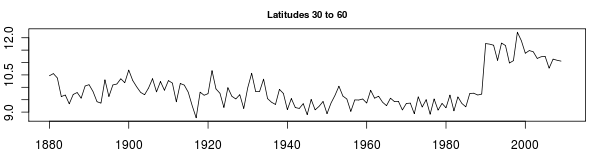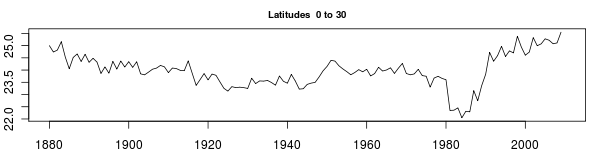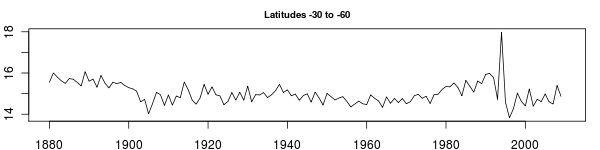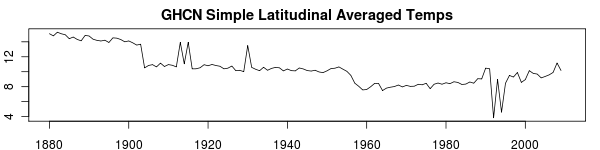We can see step changes in the last (all) chart. These are clearly related to the discontinuities in the South Polar Zone 6. And there are some strong spikes towards the end of Zone 6 that are reflected in the final average. One reason that the Zone 6 is causing so much trouble is the relative sparsity of stations there (Ranging from none with a suitable temp to a couple of dozen). Lose a few warm stations or add a few cold ones can have a big effect on that zone. So the Zone 6 is especially problematic (although 5 has some similar issues). But one of the problems is simply this: Zone 6 is 17% of the final average but Zone 6 only covers about 6.5% of the planet. Weighting the zones will help smooth this result.

1. 2010 July 11 at 1:11 pm

I’m kinda of serious about hoping for help from O’Day (or anyone else who knows how to properly set up a panel plot). There seemed to be some strange unwillingness for the xyplot to handle a group variable that was character based and not an integer. That made no sense to me since I have seen examples with groups using names. So I’m lost there.

2. 2010 July 11 at 1:40 pm

Ron,
Did you try the layout function from the graphics package? I found it works very well for panels.

3. 2010 July 11 at 9:25 pm

Thanks for the ptr, Nick.

I did try a layout and stacked six panels nicely.

But the problem was that the data shown in them was not very similar to what you see above. It also appeared that the y-range became something like 0-1000. I’ll post the data.frame and xyplot code that I tried later.

1. 2011 March 1 at 8:27 am